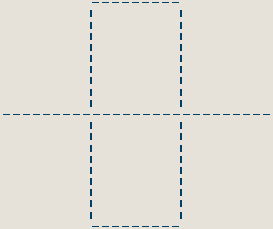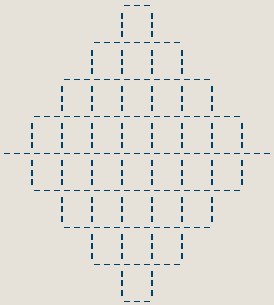David Makin's Fractal Blog

The Peano Curve:

First take a line segmentReplace the middle third with a figure of 8:Now repeat, replacing the middle third of each line segment with a figure of 8 rotated according to the orientation:If you were to continue the construction it results in a solid diamond - a 2 dimensional object.

From a topolgical point of view it's important to note that the entire construction can be created without any crossings or double-backs ie. you could draw the whole thing starting from the left end and continue to the right end without removing pen from paper or doubling back or once crossing the line (touching but not crossing) eg. for the first stage it's:
right,up,right,down,left,down,right,up,right.

The first two sections of the second stage are:
right,up,right,down,left,down,right,up,right,up,left,up,right,down,right,up,left,up.

How long would the line drawn be?
If the original line segment has unit length, then the first stage is 3 units long, the second 9, the third 27, the nth being 3^n units long so the length of the line for the infinite limit stage would be 3^infinity units! In practice the length of the line required to produce space-filling is limited by the required pixel resolution of the device used to display the image eg. if drawn with a pen or pencil then the limit length is determined by the thickness of the pen or pencil line (and the steadiness of your hand) or if the length of the original line segment on a computer display is 81 pixels then a solid diamond is produced at stage 4 and the length of the line required to draw it is 81*3^4 = 81*81 pixels.

Is it really a 2 dimensional object?
Well at each stage if we take it that we have 9 new segments of unit length then they were created from an original segment of length 3 so Measure Theory says that the fractal dimension is log(9)/log(3) = 2

This type of fractal curve (producing a 2 dimensional result) is known as a "space filling curve" and there are many of them.

Browse Galleries
Fractal Blog Archive
Art Services